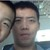# 人教版（新）数学九年级上册第二十二章二次函数测试题

• 10人评价）
• 29人阅读
• 上传者：任秋燕

### 相关文档推荐2积分 下载
```                  中国现代教育网     www.30edu.com  全国最大教师交流平台

二次函数小测试七

1、把   y= -x2-2x-3 配方成 y=a (x+m)2+n 的形式为  y=_____________
2、抛物线    y=4x2-11x-3 与 y 轴的交点坐标是_______________
3、抛物线    y= -6x2-x+2 与 x 轴的交点的坐标是___________
1
4、抛物线    y=   (x-1)2+2 的对称轴是直线__________顶点坐标为____________。
2
5、二次函数     y=ax2+bx+c，当  x=  -1 时 y=10; x=1 时 y=4  ,x=2 时   y=7 则函数解析式为

_________________.
6、二次函数     y=ax2+bx+c 的图象是抛物线，其开口方向由_________来确定。
7、方程   ax2+bx+c=0 的两根为-3，1    则抛物线     y=ax2+bx+c 的对称轴是直线____________。
8、已知   y=(k2-k) x2+kx 是二次函数，则     k 必须满足的条件是_____________________。
9、已知直线     y=2x-1 与两个坐标轴的交点是          A、B，把    y=2x2 平移后经过    A、B  两点，则平
移后的二次函数解析式为____________________

10、与抛物线     y= -x2+2x+3，关于   x 轴对称的抛物线的解析式为__________________。

1
1、抛物线    y=  (k2-2)x2+m-4kx 的对称轴是直线     x=2，且它的最低点在直线          y= -  +2 上，求
2
函数解析式。

2、二次函数     y=ax2+bx+c 的图象过点（1，0）（0，3），对称轴              x=   -1。①求函数解析式
②若图象与     x 轴交于   A、B（A    在 B 左）与   y 轴交于   C,顶点  D，求四边形      ABCD  的面
积。

2积分下载